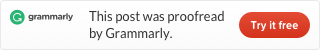# LeetCode 202. Happy Number

Write an algorithm to determine if a number is “happy”.

A happy number is a number defined by the following process: Starting with any positive integer, replace the number by the sum of the squares of its digits, and repeat the process until the number equals 1 (where it will stay), or it loops endlessly in a cycle which does not include 1. Those numbers for which this process ends in 1 are happy numbers.

Example:

```Input: 19
Output: true
Explanation:
12 + 92 = 82
82 + 22 = 68
62 + 82 = 100
12 + 02 + 02 = 1```

First, we define a function split, it can separate every digit from a number, and make a List.

Then we define a function combine，caculate the sum of the squares of all digits in the List.

Then, we keep calling split and combine until the number become 1, we return true. But sometimes we will get an infinite loop, so we put the number to a HashSet, if any number but 1 keep occurring, we quit the loop and return false.

To see more hashtable related problems, see
Problems and Solutions of Hashmap.This site uses Akismet to reduce spam. Learn how your comment data is processed.